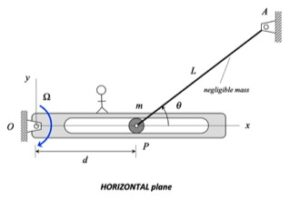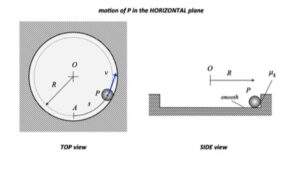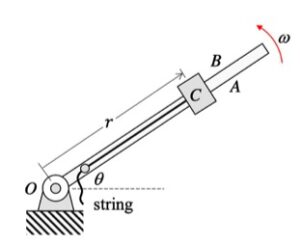# Homework H.4.L# Homework H.4.INOTE: All surfaces are smooth. Unfortunately, this information was omitted from the problem statement.

______________________

HINTS

STEP 1 - FBD: Draw a SINGLE free body diagram (FBD) of the system including Block A, Block B and the rigid bar. From this, determine which forces do non-conservative work on this system, if any.
STEP 2 - Kinetics:  Write down the work/energy equation.
STEP 3 - Kinematics: Consider the location of the instant center for bar AB. This will be the key to the kinematics that you need to solve this problem.
STEP 4 - Solve

__________________________

# Homework H.4.JHINTS

STEP 1 - FBD: Draw a SINGLE free body diagram (FBD) of the system including Block A, Block B and the cable. From this, determine which forces do work on this system.
STEP 2 - Kinetics:  Write down the work/energy equation. Determine the work done by the forces that you identified  above in STEP 1.
STEP 3 - Kinematics: Review the constrained motion kinematics from Section 1.D of the course lecture book. To this end, you will write down an expression for the length of the cable in terms of sA, sB and constants. Differentiate this expression to relate the speeds of A and B.
STEP 4 - Solve

__________________________

# Homework H.4.G# Homework H.4.H# Homework H.4.E# Homework H.4.F# Homework H.4.C# Homework H.4.D